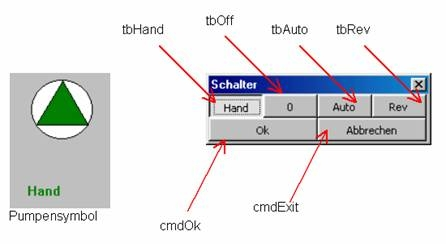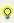Example 2 - Setting a switch (working with process variables)In this example we draw a pump consisting of a circle and a triangle. Define the triangle as a symbol. On top draw a multibinary element and link it to three bit marker variables.

Additionally define, which color the triangle should get, if the values of the variables change.

First we combine the multibinary element with a macro, which opens a form frmSwitch.

In the form frmSwitch we will be able to change the values of the three bit marker variables.Info Only one of the three variables may have the value 1. (i.e. if one variable is set to 1, the other two have to be set to 0)

To be able to use this macro several times in project with different variables, you only may link bit marker variables ti the multibinary element, which contail in their names, which status of the pump they control.

e.g.:
Variable_Auto
Variable_Hand
Variable_ReviInfo The suffixes _Auto, _Hand and _Revi are fixly defined in the source code of the example.

With this five characters suffix of the variable names it is defined, which variable is set to 1 and which is set to 0 on clicking a toggle button.

In the macro LeftClickUp_Switch a sub program FindVariable is called in the form frmSwitch, which gets the clicked element obElem.

Public Sub LeftClickUp_Schalter (obElem As Element)
frmSchalter.FindVariable obElem
position (pixel to points = (pixel * 0.75))
frmSchalter.Top = obElem.Bottom * 0.75
frmSchalter.Left = obElem.Left * 0.75
frmSchalter.Show
obElem.LeftClickUp
End Sub

Module global variable declaration:

Dim cmdLast As ToggleButton
Dim strHand As String
Dim strAuto As String
Dim strRevi As String
In the sub program FindVariable all variables linked to the passed element are checked.

Depending on the suffix (_Auto, _Hand or _Revi) the variable names are assigned to the string variables declared above.

Additionally the status of the variables is determined and depending on the value (1 or 0) the according toggle buttons are pressed or not.

On opening the form frmSwitch the name of the currently pressed toggle button is written to a string variable. For the case, that the user decides to cancel his action, the original values are reset.

Public Sub FindVariable (obElem As Element)
Dim i As Integer
Dim obVariable As Variable

For i = 0 To obElem . CountVariable - 1
Select Case Right \$( obElem . ItemVariable ( i ). Name , 5 )
Case _ Auto
strAuto = obElem . ItemVariable ( i ). Name

Case _ Hand
strHand = obElem . ItemVariable ( i ). Name

Case _ Revi
strRevi = obElem . ItemVariable ( i ). Name
End Select
Next i

Set obVariable = thisProject . Variables . Item ( strHand )
If obVariable . Value = 1 Then
tbHand . Value = True
Set cmdLast = tbHand
End If

Set obVariable = thisProject . Variables . Item ( strAuto )
If obVariable . Value = 1 Then
tbAuto . Value = True
Set cmdLast = tbAuto
End If

Set obVariable = thisProject . Variables . Item ( strRevi )
If obVariable . Value = 1 Then
tbRev . Value = True
Set cmdLast = tbRev
End If

If tbHand . Value = False And tbAuto . Value = False And tbRev . Value = False Then
tbOff . Value = True
Set cmdLast = tbOff
End If
End Sub

The self-created function VarExists only checks, whether the linked variables really exist. If this is not the case, an error message is displayed. Variable doesn't exist.

Function VarExists ()

Dim obVariable As Variable
Set obVariable = thisProject . Variables . Item (strHand)

If obVariable Is Nothing Then
MsgBox (Variable doesnt extist)
VarExitsts = False
Exit Function
End If

Set obVariable = thisProject . Variables . Item (strAuto)
If obVariable Is Nothing Then
MsgBox ( Variable doesnt extist )
VarExitsts = False
Exit Function
End If

Set obVariable = thisProject . Variables . Item (strRev)
If obVariable Is Nothing Then
MsgBox ( Variable doesnt extist )
VarExitsts = False
Exit Function
End If

VarExists = True
End Function

If the user clicks Cancel, the value change is undone and the original status is reset.

Private Sub cmdExit _ Click ()
cmdLast.Value = True
End Sub

Private Sub cmdOk _ Click ()